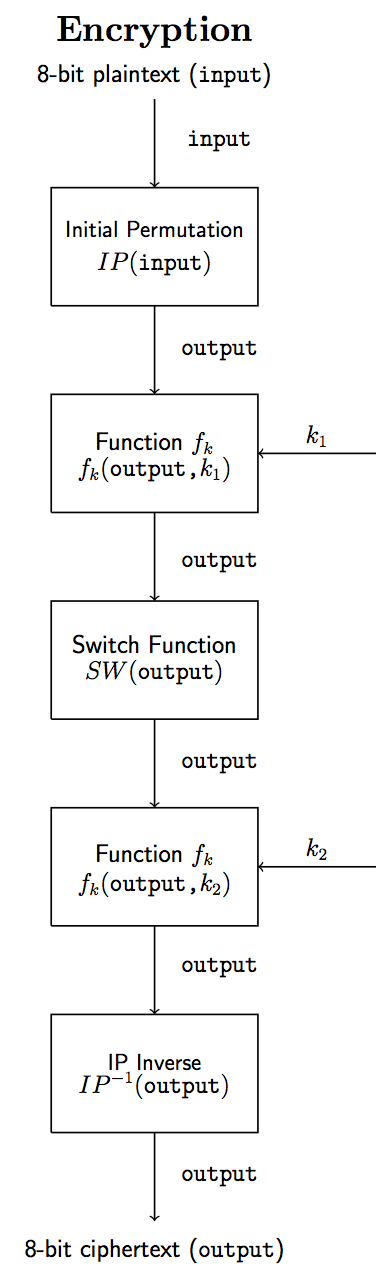# Simplified DES (SDES): an implementation in C

- 3 mins

A few months ago, I went through a quite challenging experience as a student of an introdutory class on Criptography: I was required to give a presentation on DES algorithm.

After going through the titles of the reliable books on the subject, I decided to read Criptography and Network Security by William Stallings and one of the first sentences on that page caught my attention:

“The structure of DES and most symmetric ciphers is very complex and cannot be explained as easily.”.

Therefore, in the next paragraph, it said that studying a simplified version (SDES) would enhance the understanding of DES, and I can guarantee that it actually does.

After a couple of hours struggling in the beginning, I finally got the idea behind one of the most important symmetric ciphers algorithms.

## The encryption process

The simplified DES operates on a 8-bit block of plaintext to generate a 8-bit block of ciphertext. These types of algorithms are called block ciphers. It also uses a 10-bit key, generating two subkeys in the process, used as input for the most delicate part of the algorithm, the fk function.

The scheme below shows how the plaintext (input) is manipulated to generate the ciphertext (output).After all these steps, the output is the encrypted input.

## The keys generation step

This step only involves single permutations and shifts. I strongly believe that if you take a look at the implementation you won’t have any problems understanding it.

## The decryption process

DES is a symmetric cipher, which means that it uses the same key to encrypt and decrypt the data. Since my idea was only show the step-by-step encryption process, I decided not to write the function to decrypt it.

However, you might not have any problems doing it either, because the only thing you’ll need to do is the encryption process backwards, i.e.: output -> IP(output) -> fk(output, k2) -> SW(output) -> fk(output, k1) -> IP Inverse (output) -> input.

## The implementation in C

Based on the scheme shown above, I implemented the algorithm and came up with the encrypt function, that summarizes the encryption process of Simplified DES.

To make it easier to understand, I tried to stick to the names of the variables. The variables input, key (also the subkeys k1 and k2) and output literally mean the same as on the scheme.